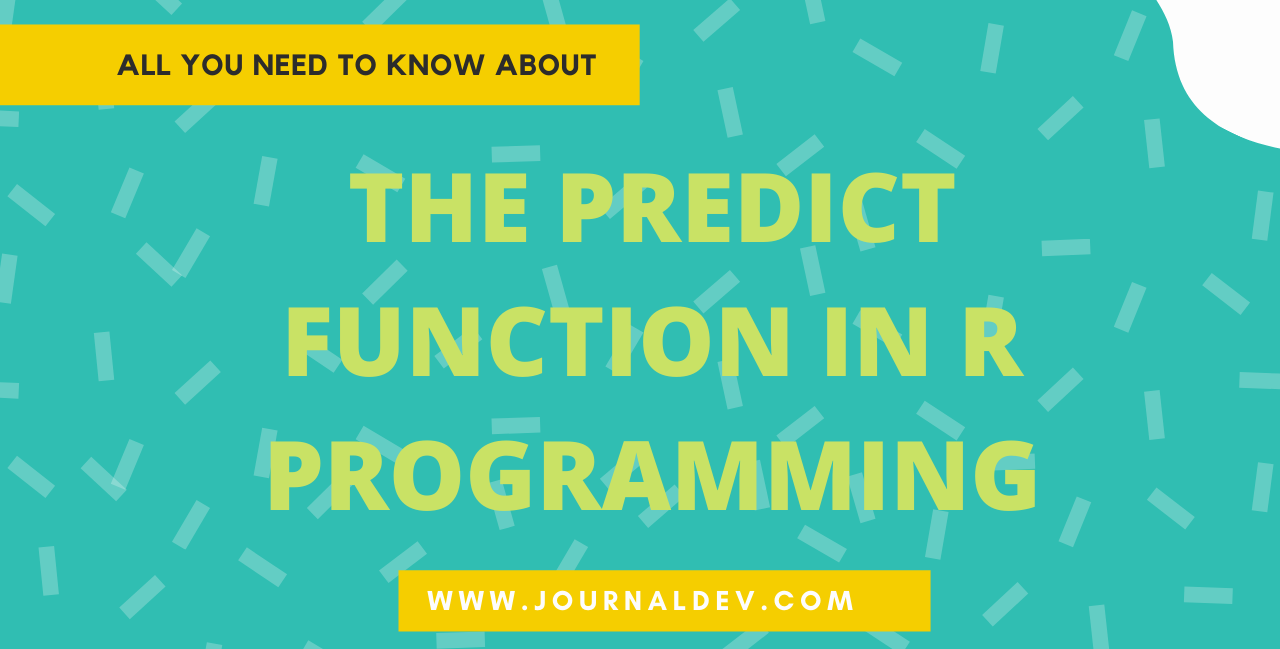# How To Use the predict() Function in R Programming

Published on August 3, 2022 · Updated on September 30, 2022By Prajwal CN### Introduction

The `predict()` function in R is used to predict the values based on the input data. All the modeling aspects in the R program will make use of the `predict()` function in their own way, but note that the functionality of the `predict()` function remains the same irrespective of the case.

In this article, you will explore how to use the `predict()` function in R.

## Prerequisites

To complete this tutorial, you will need:

## Syntax of the `predict()` function in R

The `predict()` function in R is used to predict the values based on the input data.

``````predict(object, newdata, interval)
``````
• object: The class inheriting from the linear model
• newdata: Input data to predict the values
• interval: Type of interval calculation

## An example of the predict() function

We will need data to predict the values. For the purpose of this example, we can import the built-in dataset in R - “Cars”.

``````df <- datasets::cars
``````

This will assign a data frame a collection of `speed` and distance (`dist`) values:

``````     speed dist
1      4    2
2      4   10
3      7    4
4      7   22
5      8   16
6      9   10
7     10   18
8     10   26
9     10   34
10    11   17
``````

Next, we will use `predict()` to determine future values using this data.

First, we need to compute a linear model for this data frame:

``````# Creates a linear model
my_linear_model <- lm(dist~speed, data = df)

# Prints the model results
my_linear_model
``````

Executing this code will calculate the linear model results:

``````Call:
lm(formula = dist ~ speed, data = df)

Coefficients:
(Intercept)        speed
-17.579        3.932
``````

The linear model has returned the speed of the cars as per our input data behavior. Now that we have a model, we can apply `predict()`.

``````# Creating a data frame
variable_speed <- data.frame(speed = c(11,11,12,12,12,12,13,13,13,13))

# Fiting the linear model
linear_model <- lm(dist~speed, data = df)

# Predicts the future values
predict(linear_model, newdata = variable_speed)
``````

This code generates the following output:

``````       1        2        3        4        5
25.67740 25.67740 29.60981 29.60981 29.60981
6        7        8        9       10
29.60981 33.54222 33.54222 33.54222 33.54222
``````

Well, we have successfully predicted the future distance values based on the previous data and with the help of the linear model.

Now, we have to check the “confidence” level in our predicted values to see how accurate our prediction is.

## Confidence in the Predicted Values

The confidence interval in the predict function will help us to gauge the uncertainty in the predictions.

``````# Input data
variable_speed <- data.frame(speed = c(11,11,12,12,12,12,13,13,13,13))

# Fits the model
linear_model <- lm(dist~speed, data = df)

# Predicts the values with confidence interval
predict(linear_model, newdata = variable_speed, interval = 'confidence')
``````

This code generates the following output:

``````      fit      lwr      upr
1  25.67740 19.96453 31.39028
2  25.67740 19.96453 31.39028
3  29.60981 24.39514 34.82448
4  29.60981 24.39514 34.82448
5  29.60981 24.39514 34.82448
6  29.60981 24.39514 34.82448
7  33.54222 28.73134 38.35310
8  33.54222 28.73134 38.35310
9  33.54222 28.73134 38.35310
10 33.54222 28.73134 38.35310
``````

You can see the confidence interval in our predicted values in the above output.

From this output, we can predict that the cars which are traveling at a speed of 11-13 mph have a likelihood to travel a distance in the range of 19.9 to 31.3 miles.

## Conclusion

The `predict()` function is used to predict the values based on the previous data behaviors and thus by fitting that data to the model.

You can also use the confidence intervals to check the accuracy of our predictions.

References

Thanks for learning with the DigitalOcean Community. Check out our offerings for compute, storage, networking, and managed databases.Prajwal CN

author

#### Still looking for an answer?

Ask a questionSearch for more help

JournalDev
DigitalOcean EmployeeJanuary 1, 2022

What is the meaning of attribute Fit in the above result set?

- Learner

JournalDev
DigitalOcean EmployeeSeptember 11, 2021

speed prediction calculation details please for the new learners.that table shows just the numbers . Thank You

- Learner

JournalDev
DigitalOcean EmployeeMay 20, 2021

“The output clearly says that the cars which are traveling at a speed of 11-13 mph have chances to travel the distance in the range of 19.9 to 31.3 miles.” No, it really doesn’t.

- None ya